Warning

This documents an unmaintained version of NetworkX. Please upgrade to a maintained version and see the current NetworkX documentation.

# clustering¶

clustering(G, nodes=None, weight=None)[source]

Compute the clustering coefficient for nodes.

For unweighted graphs, the clustering of a node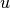is the fraction of possible triangles through that node that exist,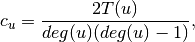where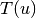is the number of triangles through nodeand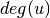is the degree of.

For weighted graphs, the clustering is defined as the geometric average of the subgraph edge weights ,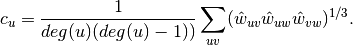The edge weights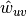are normalized by the maximum weight in the network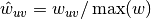.

The value of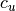is assigned to 0 if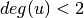.

Parameters: G (graph) – nodes (container of nodes, optional (default=all nodes in G)) – Compute clustering for nodes in this container. weight (string or None, optional (default=None)) – The edge attribute that holds the numerical value used as a weight. If None, then each edge has weight 1. out – Clustering coefficient at specified nodes float, or dictionary

Examples

>>> G=nx.complete_graph(5)
>>> print(nx.clustering(G,0))
1.0
>>> print(nx.clustering(G))
{0: 1.0, 1: 1.0, 2: 1.0, 3: 1.0, 4: 1.0}


Notes

Self loops are ignored.

References

  Generalizations of the clustering coefficient to weighted complex networks by J. Saramäki, M. Kivelä, J.-P. Onnela, K. Kaski, and J. Kertész, Physical Review E, 75 027105 (2007). http://jponnela.com/web_documents/a9.pdf